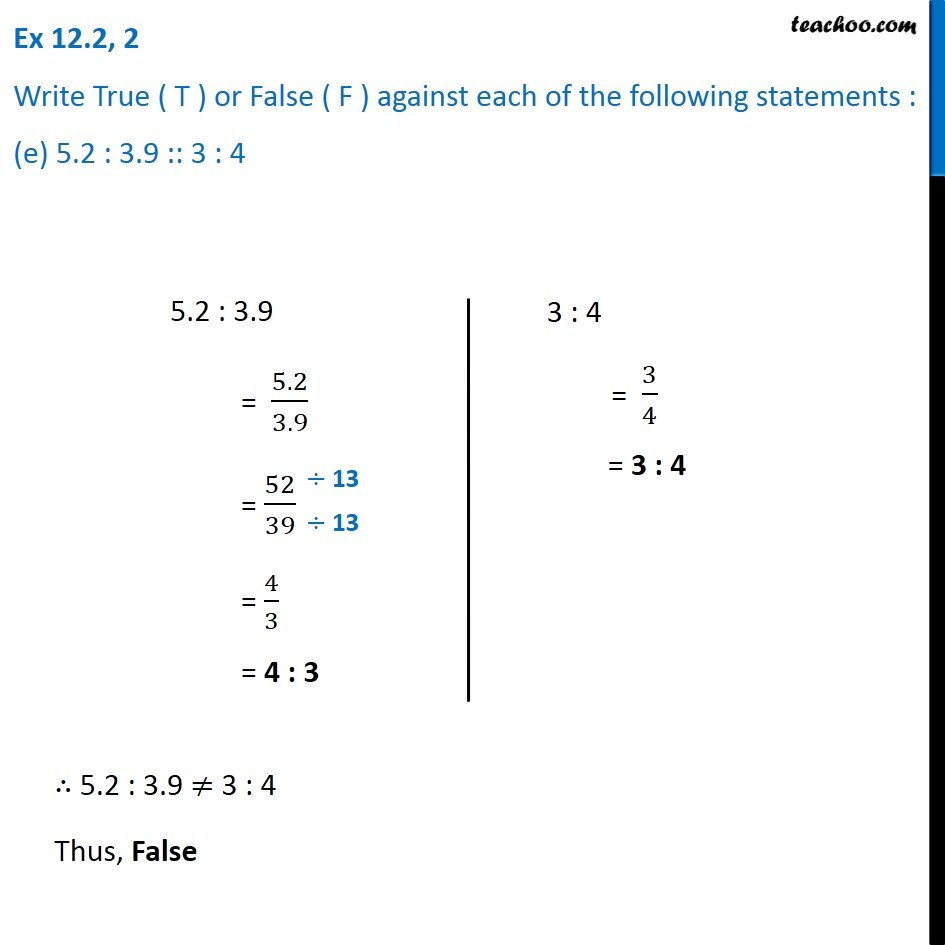1. Chapter 12 Class 6 Ratio And Proportion
2. Serial order wise
3. Ex 12.2

Transcript

Ex 12.2, 2 Write True ( T ) or False ( F ) against each of the following statements : (e) 5.2 : 3.9 :: 3 : 45.2 : 3.9 = 5.2/3.9 = 52/39 = 4/3 = 4 : 3 3 : 4 = 3/4 = 3 : 4 ∴ 5.2 : 3.9 ≠ 3 : 4 Thus, False